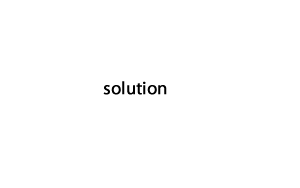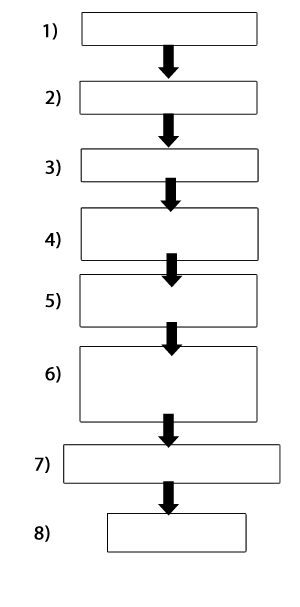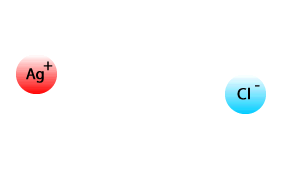Gravimetric analysis- sample calculations A 3.46 g sample of limestone(CaCO3 ) was dissolved in 0.1M (HCl) solution according to the following equation. CaCO3 (s) + 2HCl (aq) => Ca2+(aq) + 2Cl -(aq) + CO2(g) + H2O (l) Excess 0.1M (NH4)2C2O4(aq), was added to the resulting solution to precipitate the calcium ions as calcium oxalate, CaC2O4(s). The precipitate was filtered, dried and weighed at 2.03 g. Determine the percentage by mass of calcium in the limestone sample. Step 1 Write the balanced ionic equation for the precipitation reaction: Solution2) Visualise what is happening and complete the flow chart on the right with the steps below. a) Add HCl b) Crush the limestone c) Weigh the sample of limestone d) Add excess 0.1M ammonium oxalate e) Dry the precipitate f) Wash the precipitate g) Filter the precipitate h) Weigh the precipitate. SolutionStep 2 Calculate the mole of precipitate formedStep 3 Find the mole of Ca2+ ions in step 1) the ratio of Ca2+ reacting and CaC2O4 produced is 1:1 so the mol of Ca2+ is 0.0158. Step 4 Find the mass of Ca2+.Step 5 Calculate the percentage, by mass, of calcium in the original limestone sample.1) What are the properties of an ideal precipitate that can be used in gravimetric analysis. low solubility high purity its composition is always known easily filtered all of the above 2) The precipitate trapped in the filter paper is usually washed thoroughly. In this case it is to remove sodium ions remove dust remove chloride ions make the precipitate easier to filter all of the above 3) A precipitate that is not thoroughly washed tends to give results that are lower than the actual results higher than the actual results the same as the actual results all of the above 4) When washing the precipitate, a small sample of the filtrate is periodically tested with silver nitrate solution. Why? to make sure no calcium ions are coming through the filter paper and being lost. to make sure all the chloride ions have been washed off the precipitate to see if it has been contaminated by airborne particles none of the above 5) In one gravimetric analysis a precipitate of silver chloride is formed by adding silver nitrate to a sample containing chloride ions, as shown on the right. a) Which ion is the contaminant and where did it come from? the nitrate ion is the contaminant and came as a spectator ion from the silver nitrate solution. the nitrate ion is the contaminant and came as a spectator ion from dust particles floating in the air the chloride ion is the contaminant and came as a spectator ion from the silver nitrate solution. the silver ion is the contaminant and came as a spectator ion from the silver nitrate solution. all of the above b) Why can the contaminant be washed off the precipitate, without washing some of the precipitate ions away as well? c) If the precipitate was slightly soluble in water, during the washing, we would expect to to remove more of the impurities as the water and less of the precipitate ions gain mass as water molecules would attach onto the precipitate and be weighed as part of the precipitate. remove and loose some of the ions from the precipitate none of the aboveContinue with secondhand data